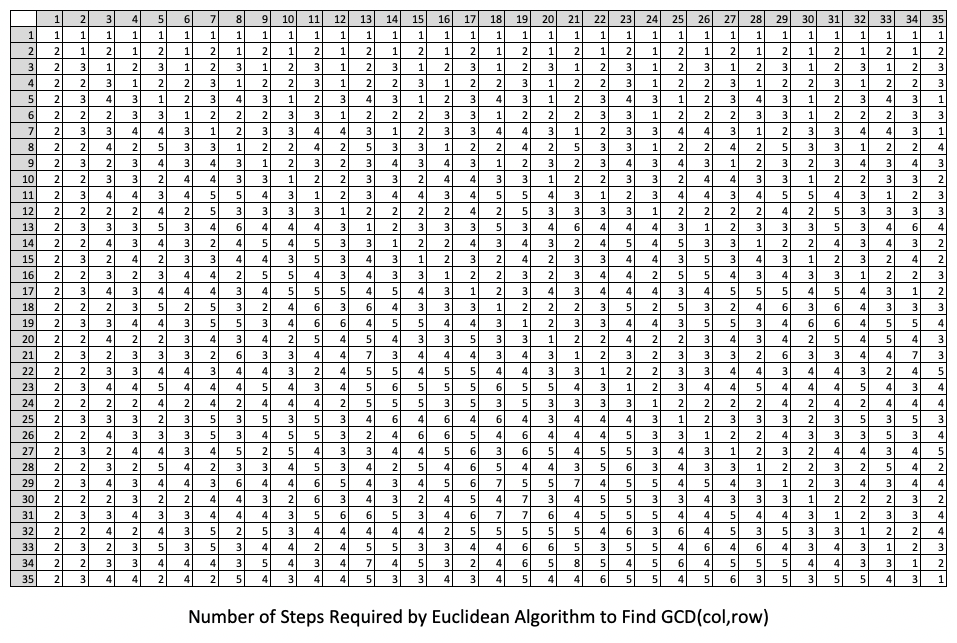## Lame's Theorem

A natural point of curiosity would be to wonder about how many steps the Euclidean Algorithm takes for a given pair of integers. As a slight variation, one might wonder what is the "smallest" pair of numbers that produces $n$ steps.

To aid in making conjectures about the above, we can appeal to technology to find the number of steps the Euclidean Algorithm takes for several pairs of integers.

Below are some calculations that one can execute in either Mathematica or R to this end:

### R

In R, we the following function will count how many steps the Euclidean Algorithm requires to compute the $gcd(m,n)$:

num.steps = function(m,n) {
cnt = 0
while (n != 0) {
m.next = n
n.next = m %% n
m = m.next
n = n.next
cnt = cnt + 1
}
return(cnt)
}


Then, we simply need to apply this to function to multiple pairs of values $(m,n)$ and record the results in a matrix -- which can be accomplished for all $0 \lt m,n \le 35$ with the following:

numrows = 35
numcols = 35
m = sapply(1:numcols,num.steps,n=1)
for (r in 2:numrows) {
m = rbind(m,sapply(1:numcols,num.steps,n=r))
}
rownames(m) <- 1:numrows
colnames(m) <- 1:numcols


Exporting this matrix to a comma-separated-values (CSV) file named "steps.csv" with write.csv(m,"steps.csv") produces a file that one can open in Excel and format to look like the table shown below:### Mathematica

We can implement the Euclidean Algorithm in Mathematica by appealing to the recursive relationship at its heart. Perhaps the shortest way to do this would be with the following:

 g[x_,y_] := Which[ Mod[x,y] == 0, y, True, g[y,Mod[x,y]] ]


Note, with a slight modification the formula above can easily keep track of how many steps the Euclidean Algorithm takes to find the gcd of two numbers:

 g[x_,y_,c_] := Which[ Mod[x,y] == 0, {y, c+1}, True, g[y, Mod[x,y], c+1] ]

Here's an example of how to call this "modified" function:

g[1160718174, 316258250, 0]

results in

{1078, 10}

which tells us that the Euclidean Algorithm took 10 steps to find the gcd of 1078.

We can create a table of how many steps different pairs of values need in the Euclidean Algorithm to compute their greatest common divisor with the following additional code:
TableBody = Table[Table[Part[g[i, j, 0], 2], {i, 35}], {j, 35}];

Now, upon looking at the table, notice the smallest positive integer $a$ for which there is a smaller number $b$ so that the Euclidean algorithm applied to $a$ and $b$ stops in $n$ steps is the $(n+2)$nd Fibonacci number. Further, the corresponding $b$ is the $(n+1)$st Fibonacci number.